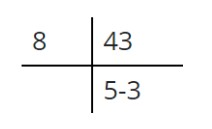# Binary to Octal Converter

Enter the binary number and click the calculate button to convert binary numbers to the octal number system.

Give Us Feedback

Binary to octal converter is used to convert the base 2 numbers into a base 8 number system.

## What is a binary number system?

A base 2 number system in which 0 and 1 numerals are used is known as a binary number system. For example, 10001 is a binary number system of a hexadecimal number 11.

## What is the octal number system?

The octal number system is a base 8 number system in which there are 8 numerals are used. The numerals of the octal number are from 0 to 7. The octal number system is indicated by the addition of an 8 suffix.

## How to convert binary to octal?

Following is a solved example of binary to octal conversion.

Example

Convert 101011 into an octal number system.

Solution

Step 1: First convert the given number from binary to decimal.

(101011)2 = (1x25) + (0x24) + (1x23) + (0x22) + (1x21) + (1x20)

(110010)2 = (1x32) + (0x16) + (1x8) + (0x4) + (1x2) + (1x1)

(110010)2 = 32 + 0 + 8 + 0 + 2 + 1

(110010)2 = 43

Step 2: Convert decimal to the octal number system.Hence, 53 is the octal number of (101011)2.

### Math Tools